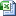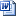# 咸宁市市场监督管理局 食品安全监督抽检信息公告 （2019年第16 期）

（一）湖北民丰和饮品有限公司生产的仙源饮用山泉水（生产日期/批号：2019-08-17），铜绿假单胞菌检测值为C=2(C1=5,C2=4,C3=0,C4=0,C5=0)CFU/250ML，标准规定为≤n=5,c=0,m=0CFU/250ML 。亚硝酸盐检测值为0.0051mg/L，标准规定为≤0.005mg/L。检验机构为湖北易安检测技术服务有限公司。

（二）通山县和天下饮品厂生产的众和天下包装饮用水（生产日期/批号：2019-08-17），大肠菌群检测值为C=2(C1=6,C2=11,C3=0,C4=0,C5=0)CFU/ML，标准规定为≤n=5,c=0,m=0CFU/ML 。检验机构为湖北易安检测技术服务有限公司。

（三）崇阳县磨刀山泉水有限公司生产的磨刀山泉（生产日期/批号：2019-08-18），大肠菌群检测值为C=2(C1=17,C2=0,C3=11,C4=0,C5=0)CFU/ML，标准规定为≤n=5,c=0,m=0CFU/ML 。检验机构为湖北易安检测技术服务有限公司。

（四）赤壁市思泉饮品有限公司生产的思泉 纯净水（生产日期/批号：2019-08-14），大肠菌群检测值为C=2(C1=13C2=0C3=8C4=0C5=0)CFU/ML，标准规定为≤n=5,c=0,m=0CFU/ML 。检验机构为湖北易安检测技术服务有限公司。

（五）赤壁市天新饮业有限公司生产的九和 饮用纯净水（生产日期/批号：2019-08-21），铜绿假单胞菌检测值为C=5(C1=多不可计，C2=210C3=157C4=320C5=198)CFU/250ML，标准规定为n=5,c=0,m=0 CFU/250ML  。检验机构为湖北易安检测技术服务有限公司。

（六）赤壁市吉山泉矿泉水有限公司生产的吉山泉（生产日期/批号：2019-08-21），铜绿假单胞菌检测值为C=2(C1=50C2=0C3=72C4=0C5=0)CFU/250ML，标准规定为n=5,c=0,m=0 CFU/250ML 。检验机构为湖北易安检测技术服务有限公司。

（七）咸宁市农雾泉纯净水厂生产的农雾泉饮用水（生产日期/批号：2019-08-21），铜绿假单胞菌检测值为C=3C1=189C21C31C4=205C5=57CFU/250ML，标准规定为n=5,c=0,m=0 CFU/250ML。检验机构为湖北易安检测技术服务有限公司。

（八）赤壁市仙人洞矿泉饮品厂生产的仙人泉 包装饮用水（生产日期/批号：2019-08-21），铜绿假单胞菌检测值为C=5C1=25C2=12C3=11C4=20C5=28CFU/250ML，标准规定为n=5,c=0,m=0 CFU/250ML。检验机构为湖北易安检测技术服务有限公司。

（九）嘉鱼亿万康纯净水厂生产的億客来 饮用纯净水（生产日期/批号：2019-08-22），铜绿假单胞菌检测值为c=5(5个样品均为多不可计）CFU/250M，标准规定为n=5,c=0,m=0CFU/250M 。检验机构为湖北易安检测技术服务有限公司。

（十）咸宁市咸安区鄂人谷饮品有限公司生产的竹海山泉（生产日期/批号：2019-09-23），铜绿假单胞菌检测值为C=5(其中：C1=41C2=78C3=33C4=19C5=28)CFU/250M，标准规定为n=5,c=0,m=0CFU/250M。检验机构为湖北易安检测技术服务有限公司。

（十一）咸宁天怡纯水有限公司生产的怡爽饮用纯净水（生产日期/批号：2019-09-27），铜绿假单胞菌检测值为C=5(其中：C1=90C2=52C3=33C4=12C5=45)CFU/250M，标准规定为n=5,c=0,m=0CFU/250M。检验机构为湖北易安检测技术服务有限公司。

（十二）通城县七里山矿泉水有限公司生产的七里山  天然矿泉水（生产日期/批号：2019-10-30），铜绿假单胞菌检测值为C=5(其中：C1=15C2=75C3=59C4=128C5=151)CFU/250ML，标准规定为n=5,c=0,m=0CFU/250ML 。检验机构为湖北易安检测技术服务有限公司。

（十三）通城县康乐滋饮品厂生产的康乐滋  纯净水（生产日期/批号：2019-10-27），铜绿假单胞菌检测值为C=5(其中：C1=15C2=45C3=97C4=59C5=130)CFU/250ML，标准规定为n=5,c=0,m=0CFU/250ML  。检验机构为湖北易安检测技术服务有限公司。附件2食品抽检不合格情况表包装饮用水.xls附件1.本次检验项目(1)市局包装饮用水.docx附件3食品抽检合格情况表包装饮用水.xls